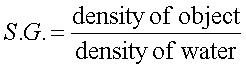### By the end of this lesson, you will be able to:

• calculate a single variable (density, mass or volume) from the density equation
• calculate specific gravity of an object, and
• determine whether an object will float or sink given its density and the density of its surroundings.

# How do I Calculate Density?Density and specific gravity in the geosciences

## An introduction to density

Density is the mass of an object divided by its volume.Density often has units of grams per cubic centimeter (g/cm3). Remember, grams is a mass and cubic centimeters is a volume (the same volume as 1 milliliter).

Density is a fundamental concept in the sciences; you will see it throughout your studies. It is used quite often in identifying rocks and minerals since the density of substances rarely changes significantly. For example, gold will always have a density of 19.3 g/cm3; if a mineral has a density other than that, it isn't gold.

You probably have an intuitive feeling for density in the materials you use often. For example, sponges are low in density; they have a low mass per unit volume. You are not surprised when a large sponge is easy to lift. In contrast, iron is dense. If you pick up an iron skillet, you expect it to be heavy.

Students, and even teachers, often confuse mass and density. The words heavy and light on their own refer to mass, and not density. A very large sponge may weigh a lot (have a high mass), but its density is low because it still weighs very little per unit of volume. For density, you also need to consider the size, or volume, of the object.

## How do I determine density?

Density is not something that is directly measured. Typically if you want to know the density of something you will weigh it and then measure its volume.

Another tricky thing about density is that you can't add densities. If I have a rock that is made up of two minerals, one with a density of 2.8 g/cm3, and one with a density of 3.5 g/cm3, the rock will have a density between 3.5 and 2.8 g/cm3, not a density of 6.3 g/cm3. This is because both the mass and the volume of the two minerals will be added, and so when they are divided to get the density the result will be between the two.

Typical densities for gasses are on the order of thousandths of grams per cubic centimeter. Liquids often have densities of about 1.0 g/cm3, and indeed, fresh water has a density of 1.0 g/cm3. Rocks often have a density around 3 g/cm3, and metals often have densities above 6 or 7 g/cm3.

## How do I calculate specific gravity?

To calculate the specific gravity (SG) of an object, you compare the object's density to the density of water:Because the density of water in g/cm3 is 1.0, the SG of an object is will be almost the same as its density in g/cm3. However, specific gravity is a unitless number, and is the same in the metric system or any other measurement system. It is very useful when comparing the density of two objects. Since specific gravity is unitless, it doesn't matter whether the density was measured in g/cm3 or in some other units (like lbs/ft3).

## Why should I calculate density or specific gravity?

Densities are critical for many uses. One of the most critical is that the density of a substance will determine if it will float on another. Less dense substances will float on (or rise through) more dense substances. Here are some examples of how this explains everyday occurrences:

A ship floating on water is a great illustration of the difference between mass and density. A ship must have a density of less than 1.0 g/cm3(the density of water), or it will sink. Ships have a large mass, because they are made of steel, but because they have a large volume, their density is less than 1.0 g/cm3. If enough mass is added to them such that their density goes above 1.0 g/cm3, they will sink.

To try some practice problems, go to the sample problem page!

## Where is density used in the geosciences?

• Isostasy - determining how high continents will sit on the mantle
• Plate tectonics - mechanisms that drive plate tectonics
• Minerals - determining the name of a mineral through its density
• Rocks - determining the name and composition of a rock by its density
• The hypsometric curve - examining the causes of elevation variation on Earth
• Oceanography - some ocean currents and ocean circulation is controlled by density

## I am ready to PRACTICE!

If you think you have a handle on all of the things listed above click on this bar to try some practice problems with worked answers!
Or, if you want even more practice, see the links below

## More help with Density

Edinformatics on-line lab on mass, volume and density is put together by NYU. It allows you to look at pictures of measurements and to enter data.

Hyperphysics, at the Georgia State has a page about density and a density converter. This includes several related pages including instructions of measuring density using the Archimedes principle.

Wikipedia's specific gravity page has an explanation of what specific gravity is and how it is used and even discusses its use in the geosciences and mineralogy. However, the content of Wikipedia articles may change and so you may want to be cautious.

Wikipedia's Density page has a general discussion of density and its history, calculation, and units. However, the content of Wikipedia articles may change and so you may want to be cautious.
This page was written and compiled by Dr. Eric M. Baer, Geology Program, Highline Community College, and Dr. Jennifer M. Wenner, Geology Department, University of Wisconsin Oshkosh